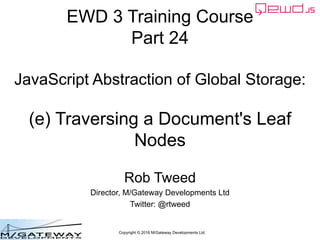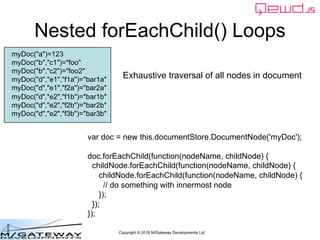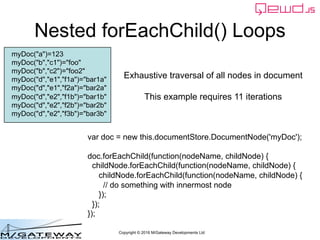Successfully reported this slideshow.

# EWD 3 Training Course Part 24: Traversing a Document's Leaf Nodes×

# EWD 3 Training Course Part 24: Traversing a Document's Leaf Nodes

This presentation is Part 24 of the EWD 3 Training Course. It examines another way to iterate through Global Storage, via its leaf nodes. In some situations this can be a faster and more efficient technique.

This presentation is Part 24 of the EWD 3 Training Course. It examines another way to iterate through Global Storage, via its leaf nodes. In some situations this can be a faster and more efficient technique.

### EWD 3 Training Course Part 24: Traversing a Document's Leaf Nodes

1. 1. Copyright © 2016 M/Gateway Developments Ltd EWD 3 Training Course Part 24 JavaScript Abstraction of Global Storage: (e) Traversing a Document's Leaf Nodes Rob Tweed Director, M/Gateway Developments Ltd Twitter: @rtweed
2. 2. Copyright © 2016 M/Gateway Developments Ltd var doc = new this.documentStore.DocumentNode('myDoc'); doc.forEachChild(function(nodeName, childNode) { childNode.forEachChild(function(nodeName, childNode) { childNode.forEachChild(function(nodeName, childNode) { // do something with innermost node }); }); }); myDoc("a")=123 myDoc("b","c1")="foo" myDoc("b","c2")="foo2" myDoc("d","e1","f1a")="bar1a" myDoc("d","e1","f2a")="bar2a" myDoc("d","e2","f1b")="bar1b" myDoc("d","e2","f2b")="bar2b" myDoc("d","e2","f3b")="bar3b" Nested forEachChild() Loops Exhaustive traversal of all nodes in document
3. 3. Copyright © 2016 M/Gateway Developments Ltd var doc = new this.documentStore.DocumentNode('myDoc'); doc.forEachChild(function(nodeName, childNode) { childNode.forEachChild(function(nodeName, childNode) { childNode.forEachChild(function(nodeName, childNode) { // do something with innermost node }); }); }); myDoc("a")=123 myDoc("b","c1")="foo" myDoc("b","c2")="foo2" myDoc("d","e1","f1a")="bar1a" myDoc("d","e1","f2a")="bar2a" myDoc("d","e2","f1b")="bar1b" myDoc("d","e2","f2b")="bar2b" myDoc("d","e2","f3b")="bar3b" Nested forEachChild() Loops Exhaustive traversal of all nodes in document This example requires 11 iterations
4. 4. Copyright © 2016 M/Gateway Developments Ltd Traversing Leaf Nodes? • Leaf Nodes are DocumentNodes that have a value and no child nodes
5. 5. Copyright © 2016 M/Gateway Developments Ltd myDoc("a")=123 myDoc("b","c1")="foo" myDoc("b","c2")="foo2" myDoc("d","e1","f1a")="bar1a" myDoc("d","e1","f2a")="bar2a" myDoc("d","e2","f1b")="bar1b" myDoc("d","e2","f2b")="bar2b" myDoc("d","e2","f3b")="bar3b" Iterating through Leaf Nodes
6. 6. Copyright © 2016 M/Gateway Developments Ltd myDoc("a")=123 myDoc("b","c1")="foo" myDoc("b","c2")="foo2" myDoc("d","e1","f1a")="bar1a" myDoc("d","e1","f2a")="bar2a" myDoc("d","e2","f1b")="bar1b" myDoc("d","e2","f2b")="bar2b" myDoc("d","e2","f3b")="bar3b" Iterating through Leaf Nodes
7. 7. Copyright © 2016 M/Gateway Developments Ltd myDoc("a")=123 myDoc("b","c1")="foo" myDoc("b","c2")="foo2" myDoc("d","e1","f1a")="bar1a" myDoc("d","e1","f2a")="bar2a" myDoc("d","e2","f1b")="bar1b" myDoc("d","e2","f2b")="bar2b" myDoc("d","e2","f3b")="bar3b" Iterating through Leaf Nodes
8. 8. Copyright © 2016 M/Gateway Developments Ltd myDoc("a")=123 myDoc("b","c1")="foo" myDoc("b","c2")="foo2" myDoc("d","e1","f1a")="bar1a" myDoc("d","e1","f2a")="bar2a" myDoc("d","e2","f1b")="bar1b" myDoc("d","e2","f2b")="bar2b" myDoc("d","e2","f3b")="bar3b" Iterating through Leaf Nodes
9. 9. Copyright © 2016 M/Gateway Developments Ltd myDoc("a")=123 myDoc("b","c1")="foo" myDoc("b","c2")="foo2" myDoc("d","e1","f1a")="bar1a" myDoc("d","e1","f2a")="bar2a" myDoc("d","e2","f1b")="bar1b" myDoc("d","e2","f2b")="bar2b" myDoc("d","e2","f3b")="bar3b" Iterating through Leaf Nodes Just 8 iterations to exhaustively traverse the example
10. 10. Copyright © 2016 M/Gateway Developments Ltd myDoc("a")=123 myDoc("b","c1")="foo" myDoc("b","c2")="foo2" myDoc("d","e1","f1a")="bar1a" myDoc("d","e1","f2a")="bar2a" myDoc("d","e2","f1b")="bar1b" myDoc("d","e2","f2b")="bar2b" myDoc("d","e2","f3b")="bar3b" Iterating through Leaf Nodes Just 8 iterations to exhaustively traverse the example We can use another DocumentNode Method: forEachLeafNode()
11. 11. Copyright © 2016 M/Gateway Developments Ltd myDoc("a")=123 myDoc("b","c1")="foo" myDoc("b","c2")="foo2" myDoc("d","e1","f1a")="bar1a" myDoc("d","e1","f2a")="bar2a" myDoc("d","e2","f1b")="bar1b" myDoc("d","e2","f2b")="bar2b" myDoc("d","e2","f3b")="bar3b" Iterating through Leaf Nodes var doc = new this.documentStore.DocumentNode('myDoc'); doc.forEachLeafNode(function(value, leafNode) ; console.log(value); });
12. 12. Copyright © 2016 M/Gateway Developments Ltd myDoc("a")=123 myDoc("b","c1")="foo" myDoc("b","c2")="foo2" myDoc("d","e1","f1a")="bar1a" myDoc("d","e1","f2a")="bar2a" myDoc("d","e2","f1b")="bar1b" myDoc("d","e2","f2b")="bar2b" myDoc("d","e2","f3b")="bar3b" Iterating through Leaf Nodes var doc = new this.documentStore.DocumentNode('myDoc'); doc.forEachLeafNode(function(value, leafNode) ; console.log(value); }); 123 foo foo2 bar1a bar2a bar1b bar2b bar3b
13. 13. Copyright © 2016 M/Gateway Developments Ltd myDoc("a")=123 myDoc("b","c1")="foo" myDoc("b","c2")="foo2" myDoc("d","e1","f1a")="bar1a" myDoc("d","e1","f2a")="bar2a" myDoc("d","e2","f1b")="bar1b" myDoc("d","e2","f2b")="bar2b" myDoc("d","e2","f3b")="bar3b" Iterating through Leaf Nodes var doc = new this.documentStore.DocumentNode('myDoc'); doc.forEachLeafNode(function(value, leafNode) ; console.log(value); }); Leaf Node's Value
14. 14. Copyright © 2016 M/Gateway Developments Ltd myDoc("a")=123 myDoc("b","c1")="foo" myDoc("b","c2")="foo2" myDoc("d","e1","f1a")="bar1a" myDoc("d","e1","f2a")="bar2a" myDoc("d","e2","f1b")="bar1b" myDoc("d","e2","f2b")="bar2b" myDoc("d","e2","f3b")="bar3b" Iterating through Leaf Nodes var doc = new this.documentStore.DocumentNode('myDoc'); doc.forEachLeafNode(function(value, leafNode) ; console.log(value); }); DocumentNode Object for the Leaf Node
15. 15. Copyright © 2016 M/Gateway Developments Ltd myDoc("a")=123 myDoc("b","c1")="foo" myDoc("b","c2")="foo2" myDoc("d","e1","f1a")="bar1a" myDoc("d","e1","f2a")="bar2a" myDoc("d","e2","f1b")="bar1b" myDoc("d","e2","f2b")="bar2b" myDoc("d","e2","f3b")="bar3b" Iterating through Leaf Nodes var doc = new this.documentStore.DocumentNode('myDoc'); doc.forEachLeafNode(function(value, leafNode) ; // how to get the properties/subscripts array for the node? });
16. 16. Copyright © 2016 M/Gateway Developments Ltd myDoc("a")=123 myDoc("b","c1")="foo" myDoc("b","c2")="foo2" myDoc("d","e1","f1a")="bar1a" myDoc("d","e1","f2a")="bar2a" myDoc("d","e2","f1b")="bar1b" myDoc("d","e2","f2b")="bar2b" myDoc("d","e2","f3b")="bar3b" Iterating through Leaf Nodes var doc = new this.documentStore.DocumentNode('myDoc'); doc.forEachLeafNode(function(value, leafNode) ; console.log(JSON.stringify(leafNode._node.subscripts)); });
17. 17. Copyright © 2016 M/Gateway Developments Ltd myDoc("a")=123 myDoc("b","c1")="foo" myDoc("b","c2")="foo2" myDoc("d","e1","f1a")="bar1a" myDoc("d","e1","f2a")="bar2a" myDoc("d","e2","f1b")="bar1b" myDoc("d","e2","f2b")="bar2b" myDoc("d","e2","f3b")="bar3b" Iterating through Leaf Nodes var doc = new this.documentStore.DocumentNode('myDoc'); doc.forEachLeafNode(function(value, leafNode) ; console.log(JSON.stringify(leafNode._node.subscripts)); }); ['a'] ['b', 'c1'] ['b','c2'] ['d', 'e1', 'f1a'] ['d', 'e1', 'f2a'] ['d', 'e2', 'f1b'] ['d', 'e2', 'f2b'] ['d', 'e2', 'f3b']
18. 18. Copyright © 2016 M/Gateway Developments Ltd myDoc("a")=123 myDoc("b","c1")="foo" myDoc("b","c2")="foo2" myDoc("d","e1","f1a")="bar1a" myDoc("d","e1","f2a")="bar2a" myDoc("d","e2","f1b")="bar1b" myDoc("d","e2","f2b")="bar2b" myDoc("d","e2","f3b")="bar3b" Terminating Early var doc = new this.documentStore.DocumentNode('myDoc'); doc.forEachLeafNode(function(value, leafNode) ; if (leafNode._node.subscripts === 'd') return true; console.log(value); }); return true from the callback function to terminate prematurely
19. 19. Copyright © 2016 M/Gateway Developments Ltd myDoc("a")=123 myDoc("b","c1")="foo" myDoc("b","c2")="foo2" myDoc("d","e1","f1a")="bar1a" myDoc("d","e1","f2a")="bar2a" myDoc("d","e2","f1b")="bar1b" myDoc("d","e2","f2b")="bar2b" myDoc("d","e2","f3b")="bar3b" Iterating through Leaf Nodes var doc = new this.documentStore.DocumentNode('myDoc'); doc.forEachLeafNode(function(value, leafNode) ; console.log(value); }); Much faster than nested loops if you need to exhaustively traverse every node in a large, deepy-nested Document